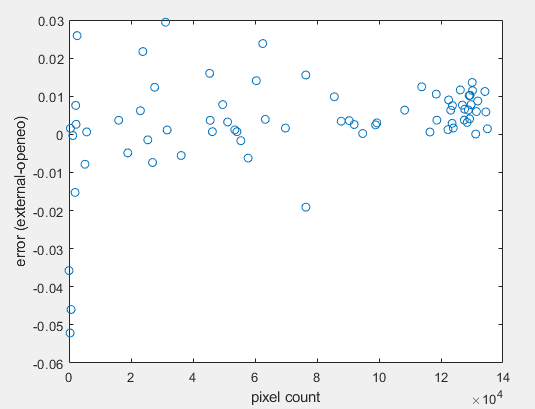# Issue with spatial mean operation

Hi,
I wanted to extract the spatial average of a cloud-masked Sentinel-2 datacube, and I noticed several issues. I decided to report them below for your knowledge:

1. When the average is downloaded as csv file, the obtained values need to be sorted, since the time variable is not consecutive
2. if the average is operated through the instruction datacube.aggregate_spatial(rect, lambda pixels: array_create([mean(pixels)])) it is possible to download the results with both netcdf and csv, while if the average is obtained through datacube.aggregate_spatial(rect, “mean”) just the csv file can be downloaded
3. The two instructions give the same results, but if the average is performed externally by downloading the full datacube the results are different:I don’t know the cause of the variability of the results. maybe there is an issue in the management of the boundary pixels?

Below is the code I used

``````# Import required packages
import openeo
from openeo.processes import process
from openeo.processes import gte, is_nan, eq, not_, sd, lte, sqrt, power, mean, array_create, quantiles
import numpy as np
from netCDF4 import Dataset
import time
import csv

# %% Initialization
#connection = openeo.connect("https://openeo.cloud")
connection = openeo.connect("https://openeo-dev.vito.be")
connection.authenticate_oidc()

# Input variables
t0= "2016-10-01"
s0="2017-10-01"

temp_ext=[t0,s0]

spat_extent={"west": 11.577, "south": 44.871, "east": 11.617, "north": 44.911}
rect={
"type":"FeatureCollection",
"features":[
{
"type":"Feature",
"geometry": {
"type":"Polygon",
"coordinates":[[[11.577,44.871],[11.577,44.911],[11.617,44.911],[11.617,44.871],[11.577,44.871]]]
},
"properties":{"name":"area1"}
}
]
}
band=["B08"]
filt= (np.ones([5,5])/25).tolist()

# S2 datacube
datacube = connection.load_collection(collection_id = "SENTINEL2_L1C_SENTINELHUB", spatial_extent = spat_extent, temporal_extent = temp_ext, bands = band,properties={"eo:cloud_cover": lambda x: lte(x, 70)}).linear_scale_range(0, 10000, 0, 1)

mask0 = connection.load_collection(collection_id = "SENTINEL2_L2A_SENTINELHUB", spatial_extent = spat_extent, temporal_extent = temp_ext, bands = maskband,properties={"eo:cloud_cover": lambda x: lte(x, 70)})

# %% try average

datacube2=datacube.aggregate_spatial(rect, lambda pixels: array_create([mean(pixels)]))

datacube2=datacube.aggregate_spatial(rect, "mean")
``````

Hi Paolo,
we’re having a two day meeting with openEO devs, so a bit slow to respond, apologies!
The most common reason for this happening is when there’s a very low number of valid pixels.
You could try counting the pixels to determine if this can be the explanatin. Not able to test directly, but I think this should work:

``````array_create([mean(pixels),count(pixels])
``````

In case you’re not able to experiment further yourself, we can try to look into this somewhere next week!

best regards,
Jeroen

hi @jeroen.dries,
I performend the calculation.The pixel count seems to have an effect, since the greatest (negative) errors correspond witht the lowest count values, but the noise is also present when many valid pixels are available…
Therefore there could be more than one explanation…